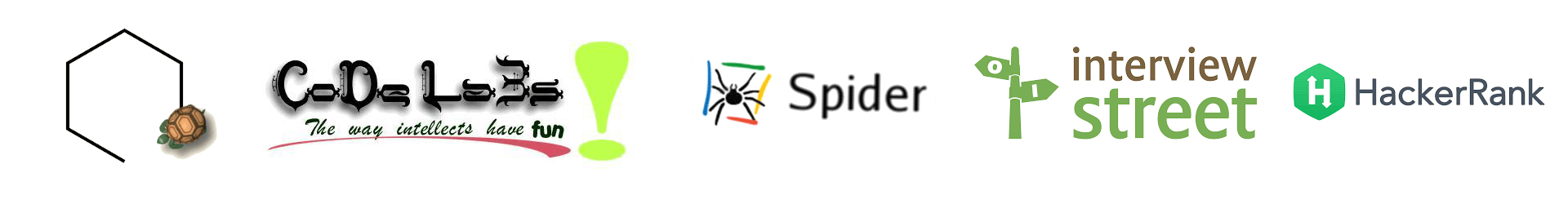I see, learn and rediscover… everyday!# Obfuscate C Code – Part III

Hi all,

This is my third post on the series of articles I’m writing on C code obfuscation. You can check all the posts I’ve written about obfuscation here.

The task for this post is quite simple. Given two numbers a and b (b>a>3) as input, print all prime numbers in between a and b (including a and b).

Let me first paste final code here. I’ll be happy if you feel that you can’t make head or tail of it. 😉

``` #define _\$_ "%d",&I);I;}) Y,A,Z,H,I;main() { #define __\$__ ({ int I; scanf(_\$_ #define FOR(_,__,___)for(_=__;_<___;+_++) Y=__\$__,A=__\$__;FOR(Z, #define T ({sscanf("2 is the only even prime number. Do you know that?", _\$_ Y,A+T/2)FOR(H,T,Z)if(!(Z%H)||(H==Z-1&&printf("%d\n",Z)))break;} ```

Like the last post, we will start with a simple, clean code. The following code solves the given task.

``` #include <stdio.h> int main() {     int a, b, i, j;     scanf("%d", &a);     scanf("%d", &b);     for (i=a ; i<=b; i++) {         for (j=2; j<i; j++) {             if (i%j == 0) {                 break;             }             else if (j==i-1) {                 printf("%d\n", j+1);             }         }     }     return 0; } ```

First thing we see here is that we have two for loops. We can replace the for loops using preprocessor statement. This is a common time saving technique if you participate in online programming contests like Topcoder or SPOJ. Also let us remove {} for single line statements.

``` #define FOR(i,a,b) for(i=a;i<b;+i++) int main() {     int a, b, i, j;     scanf("%d", &a);     scanf("%d", &b);     FOR(i, a, b+1)         FOR(j, 2, i) {             if (i%j == 0) break;             else if (j==i-1 && printf("%d\n", i));         }     return 0; }```

Next step, we remove the return 0 statement and modify int main as simply main. Also we change all the integer variables we use in our code as global variables so that we need not defing their data type. Another modification that can be done here is to replace 2 by TWO and have a preprocessor statement to define TWO. This preprocessor statement is placed in between the for statement, just before we need the value 2.

Next modification is to is to remove the if else and have it in a single if statement. The idea used here is short-circuiting, so that there is no need for the else statement.

``` a,b,i,j,t;main(){ scanf("%d",&a); scanf("%d",&b); #define FOR(i,a,b) for(i=a;i<b;+i++) FOR(i,a,b+1) FOR(j, #define TWO 2 TWO,i) if (i%j==0||(j==i-1&&printf("%d\n",i)))break;} ```

Next we see that #define TWO 2 is straight forward. So modify that as shown below. The catch here is that you can have any statement which starts with 2 and has any non digit character following the 2. That way sscanf will just store the value of 2 to TWO.

``` a,b,i,j,t; main(){ scanf("%d",&a); scanf("%d",&b); #define FOR(i,a,b) for(i=a;i<b;+i++) FOR(i, #define TWO ({sscanf("2 is the only even prime number. Do you know that?", "%d",&t);t;}) a,b+1) FOR(j,TWO,i) if(!(i%j)||(j==i-1&&printf("%d\n",i)))break;} ```

Finally, we see that we have 2 scanfs and 1 sscanf. The tail part of these functions are similar. Like
scanf(“%d”,&a);
scanf(“%d”,&b);
sscanf(“2 is the only even prime number. Do you know that?”, “%d”,&t);

In the above three statements, we see that “%d”, &variable); is common. So add one preprocessor statement to replace that as shown below. Rename the variables to single characters. I have used upper case here. ;-).

``` #define _\$_ "%d",&I);I;}) Y,A,Z,H,I;main() { #define __\$__ ({ int I; scanf(_\$_ #define FOR(_,__,___)for(_=__;_<___;+_++) Y=__\$__,A=__\$__;FOR(Z, #define T ({sscanf("2 is the only even prime number. Do you know that?", _\$_ Y,A+T/2)FOR(H,T,Z)if(!(Z%H)||(H==Z-1&&printf("%d\n",Z)))break;} ```

You can download all the C files as a single zip file here.

1.vivek

I could only see some hearts, no head/tail 😉

2.sp2hari

lol lol 🙂 I guess then this code has accomplished it’s mission. 😀
Trust me, this code was written only for that 😀

3.XTRobot

Dude, where did you learn so much about programming, algorithms and data structures, can you recommend some book ?

Thank you 🙂

This site uses Akismet to reduce spam. Learn how your comment data is processed.